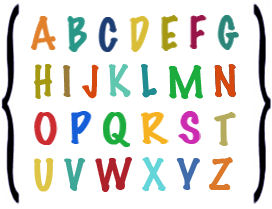# Types of Sets

## Learn About Sets With The Following Examples And Interactive ExercisesWe learned how to write sets using roster notation, as shown in examples 1 and 2 below.

Example 1: Let R be the set of all vowels in the English alphabet. Describe this set using roster notation.

Solution: R = {a, e, i, o, u}

Example 2: Let S be the set of all letters in the English alphabet. Describe this set using roster notation.

Solution: S = {a, b, c, d, e, f, g, h, i, j, k, l, m, n, o, p, q, r, s, t, u, v, w, x, y, z}

In example 2, there are 26 elements in set S. It would be easier to use a shortcut to list this set:

Example 2: Let S be the set of all letters in the English alphabet. Describe this set using roster notation.

Solution: S = {a, b, c, ..., x, y, z}

The three dots are called an ellipsis. We use an ellipsis in the middle of a set as a shortcut for listing many elements. Note that the number of elements in set and set S is countable, So each of these sets is a finite set. A finite set has a finite number of elements. Let's examine another type of set:

Example 3: Let T be the set of all whole numbers.

Solution: T = {0, 1, 2, 3, 4, 5, 6, ...}

In example 3, we used an ellipsis at the end of the list to indicate that the set goes on forever. Set T is an infinite set.  An infinite set is a set with an infinite number of elements. It is not possible to explicitly list out all the elements of an infinite set. Let's look at some more examples of finite and infinite sets.

 F I N I T E  S E T S I N F I N I T E  S E T S Description Roster Notation Description Roster Notation A = {whole numbers between 0 and 100} A = {1, 2, 3, ..., 97, 98, 99} W = {even whole numbers} W = {0, 2, 4, 6, 8, ...} B = {primary colors} B = {red, blue, yellow} X = {atoms in the universe} X = {atom1, atom2, atom3, ...} C = {prime numbers less than 12} C = {2, 3, 5, 7, 11} Y = {prime numbers} Y = {2, 3, 5, 7, 11, ...}

The ellipsis makes it easier to list both finite and infinite sets with roster notation. There are some sets that do not contain any elements at all, as shown below.

Example 4: Let D be the set of all weeks with 8 days.

Solution: D = {}

We call a set with no elements the null or empty set. It is represented by the symbol { } or Ø . So = {} or = Ø. Let's look at some more examples of empty sets.

 Empty (Null) Sets Description Notation The set of dogs with sixteen legs. X = {} The set of computers that are both on and off. Y = {} The set of triangles with 4 sides. Z = {} The set of months with 32 days. D = Ø The set of bicycles with no wheels. E = Ø The set of whole numbers that are odd and even. F = Ø

Summary: An ellipsis is a shortcut used when listing sets with roster notation. A finite set has a countable number of elements: An infinite has an infinite number of elements, such as the set of whole numbers, which goes on forever. We call a set with no elements the null or empty set.

Exercises